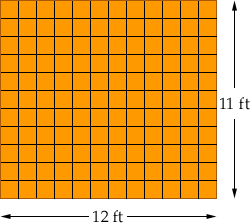Name: Amber Who is asking: Student Level: Middle Question: i have a question concerning math i have to calculate the square footage of carpet for me room and im not quite sure how to do that. the carpet is \$2.08 per sq. ft. and my room is 12x11ft. thanx! Hi Amber, Think of the flooring as one foot square tiles rather than a carpet.How many one foot square tiles are there? If each tile cost \$2.08, how much would the flooring cost? Cheers, Penny Go to Math Central